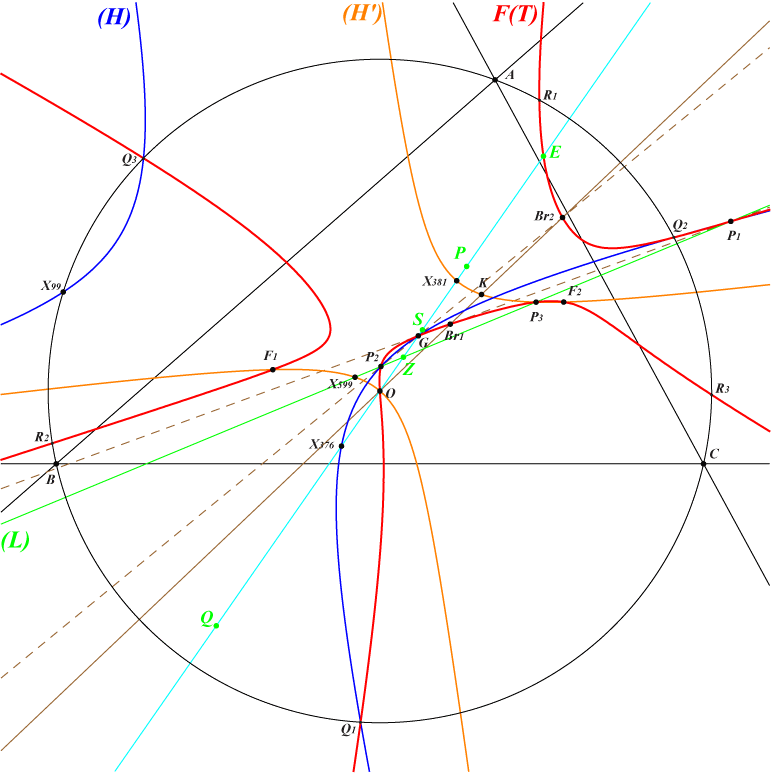The Thomson cubic K002 and its Thomson-isogonal transform K758 have nine known common points namely X(2), X(3), the four foci X(39162), X(39163), X(39164), X(39165) of the Steiner inellipse and the vertices Q1, Q2, Q3 of the Thomson triangle (T). See Table 56 for related curves. They generate a pencil of cubics passing through these same nine points which is stable under Thomson-isogonal conjugation. Two cubics are globally invariant namely K463 (an isogonal focal nK in (T)) and K1259 (an isogonal pK in (T)). The pencil contains the stelloid K833 and its Thomson-isogonal transform K727. K1329 and K1330 are two other remarkable cubics of the pencil. *** Every cubic F(T) of the pencil can be written F(T) = 16 ∆^2 K002 + T K463, where ∆ is the area of ABC and T a real number or a degree 0 expression in a, b, c. Obviously, F(0) is K002 and F(∞) is K463. The Thomson-isogonal transform of F(T) is F(4/3 - T), showing that K463 and K1259 = F(2/3) are globally invariant. Properties • F(T) meets the line at infinity at the same points as a pK of the Euler pencil, namely K(P) = pK(X6, P), where P is the homothetic of O under h(G, 3T). These two cubics share the same asymptotes and meet again at three points on the Brocard axis, one of them being O and the remaining two points Br1, Br2 being inverse in the Brocard circle. These two points are imaginary when 0 < T < 4/3. They coincide in X(6) when T = 0 and in X(3) when T = 4/3. • F(T) meets the Euler line at G, O and a third point E which is the homothetic of O under h(H, T). • F(T) meets the circumcircle (O) at Q1, Q2, Q3 and three other points R1, R2, R3 which lie on a nK0(X6, R) with R on the line GK. The tangents at Q1, Q2, Q3 to F(T) concur when T = 0 , T = 2/3 and T = 4/3 giving the cubics K002, K1259 and K758. The antipodes of R1, R2, R3 on (O) lie on another pK of the Euler pencil, namely K(Q) = pK(X6, Q), where Q is the homothetic of O under h(G, 4 - 3T). Q is in fact the reflection of P about X(376). For every T, the Kiepert parabola is inscribed in the triangle R1R2R3 and the tangents at R1, R2, R3 to F(T) concur at a point M(T), hence F(T) is a psK with respect to R1R2R3. It becomes a pK when T = 0 and T = 1 giving the cubics K002 and K727, in which case M(0) = X(6) and M(1) = X(2). Then, the triangle R1R2R3 is ABC and the CircumTangential triangle respectively. M(T) = a^2(16 ∆^2 + 2(SA(2SA - 3a^2) + b^2 c^2) T + 3(c^2 - a^2)(a^2 - b^2) T^2) : : . More generally, the locus of M(T) is a hyperbola passing through X(2), X(6), X(110), X(5085), X(11284), X(14982), X(26864), X(32227). Its center is the midpoint of X(110) and X(11284), also on the lines {30, 113}, {110, 5050}, {5609, 5651}, {5650, 51522}, {6593, 9027}. • F(T) meets the perpendicular bisectotrs of ABC at O and three pairs of points which lie on a pK of the Euler pencil, namely K(S) = pK(X6, S), where S is the homothetic of O under h(G, T / (3 - 2T)). These points are the vertices of the isosceles Kiepert triangles as seen in the Euler pencil page. Note that the two cubics share the same tangent and the same polar conic at O, this latter curve being a rectangular hyperbola passing through X(3), X(6), X(2574), X(2575). See the two examples K1331 and K1332 related to the Vecten and Fermat triangles. See also K1333 related to Brocard triangles and K1334 related to centers of the Neuberg circles. • F(T) meets the Thomson-Jerabek hyperbola at X(2), X(3), Q1, Q2, Q3 and a sixth point J(T) on the line X(2), M(T). J(T) = a^2(16 ∆^2 + 6 SA (2 SA - a^2) T + 9(c^2 - a^2)(a^2 - b^2) T^2) : : . • F(T) meets the Thomson isogonal transform (H) of the Brocard axis at X(2), Q1, Q2, Q3 and two points P1, P2 on a line (L) passing through X(599) which meets the Euler line at Z homothetic of O under h(G, 3T / (3T - 2)). (H) is the rectangular hyperbola with center X(2482) passing through Q1, Q2, Q3 and X(2), X(99), X(376), X(551), X(3413), X(3414), X(5463), X(5464), etc. These two points are imaginary when 0 < T < 4/3. They lie on the two lines passing through G and Br1, Br2. • F(T) meets the line (L) at a third point P3 which lies on the rectangular hyperbola (H'), with center G, passing through the four foci of the Steiner inellipse and X(3), X(6), X(381), X(599), X(2574), X(2575), etc. (H') is the polar conic of X(30), X(3), X(6) in K003, K005, K018 respectively.